Sections

Visualize
Tree operations

Algorithm
Visualization

Homepage

## Binary Search Trees

Binary search trees store collections of items that can be ordered, such as integers. Binary search trees support the following standard operations.

• search(x): determines is an item x contained in the tree (and if so returns the item).
• insert(x): adds item x to the collection stored in the tree, if it is not already there.
• delete(x): removes item x from the collection stored in the tree.

Data structures supporting these operations are called Dictionaries. A simple implementation is to store the items in sorted order in an array. Search(x) is simply a binary search. However, insert(x) and delete(x) are inefficient as, in general, they may require many items to be shifted.

### Data Structure

A potentially more efficient implementation is provided by a binary search tree. There, some item, is stored at the root, y say. Smaller items are organized recursively in the left subtree, larger items in the right subtree: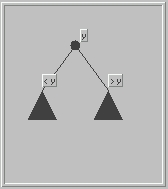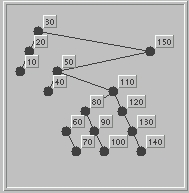For a given collection of elements, there are many possible search trees. This illustration shows a search tree with 15 nodes. It's a useful exercise to convince oneself that the search tree property stated above holds at every node.

### Search(x)

The implementation of search(x) is straight forward. We start at the root of the tree.

• Case 1 the tree is empty: then x is not present.
• Case 2 x = y (the item at the root): then the item y, at the root is returned
• Case 3 x < y : recursively search the left subtree.
• Case 4 x > y : recursively search the right subtree.
See a visualization.

In the description of insert(x) and delete(x) it is convenient to refer to a node by the item it stores; thus y means item y and the node storing y, according to the context; we may write node y to avoid ambiguity.

### Insert(x)

Insert(x) is also simple. See a visualization.

• Step 1: search(x)
• Step 2: If x is already in the tree then the insert is already done. Otherwise, the search has found a node z say, and node z has at least one empty subtree; further the search for x continued in this empty subtree, unsuccessfully, of cause.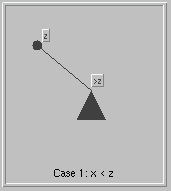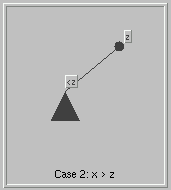In each case, a new node storing x is added as the appropriate child of z: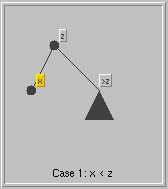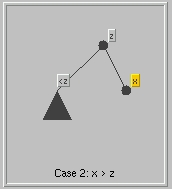### Delete(x)

Delete(x) is a little less straightforward. See a visualization.

• Step 1: search(x)
if x is not in the tree, the operation is already done. Otherwise, the goal of steps 2 and 3 is to move item x to a node with only one child.
If node x is a leaf or has just one child continue with step 4. Otherwise,
• Step 2: locate w, the predecessor of x.
w is stored at the following location:
The rightmost descendant of the left child v of x. To get there, follow right child links from v until a node with no right child is reached; this is node w (it may be that v = w).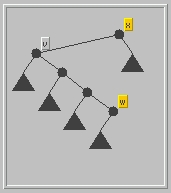• Step 3: Swap items x and w. Temporarily, the data structure is no longer a binary search tree (why?).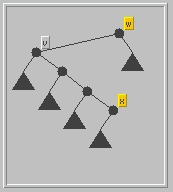• Step 4: Remove node x.
Simply replace x and its single subtree by the subtree. We need to consider the case for a single right subtree and a single left subtree separately. Our illustrations show x as a right child of p(x); however, there are cases where x is a child child.

• Case 1: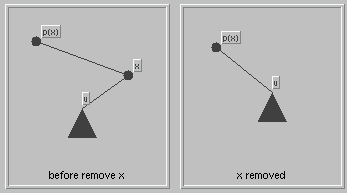• Case 2:The case in which x is a leaf is particularly simple and is left to the reader.

### References

 Data Structures and Their Algorithms, Lewis and Denenberg, Harper Collins, 1991, pp 243-251.
 "Self-adjusting Binary Search Trees", Sleator and Tarjan, JACM Volume 32, No 3, July 1985, pp 652-686.
 Data Structure and Algorithm Analysis, Mark Weiss, Benjamin Cummins, 1992, pp 119-130.
 Data Structures, Algorithms, and Performance, Derick Wood, Addison-Wesley, 1993, pp 367-375

Henning Biermann, biermann@cs.nyu.edu
Revised: March 22, 1998